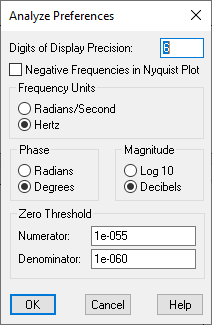### Correcting numerical round-off errors

Zeros and poles are computed by converting the linearized numerator and denominator polynomials to square matrices and then computing the corresponding eigenvalues. In general, this method is reliable and robust; however, to compensate for numerical round-off error (a floating-point number only holds 15 digits of precision), you may want to clean the numerator and denominator polynomials prior to computing the poles and zeros. This is because very small terms in the transfer function (for example, 1e-15) will become very large terms after a matrix inverse (part of the operation of finding eigenvalues), resulting in erroneously large roots or poles. To fix the problem, set a threshold value under which Embed will treat a coefficient as zero. You can set thresholds for the numerator or denominator polynomial, or the eigenvalues themselves.

To correct the polynomials

1.    Choose Analyze > Preferences.2.    In the Digits Of Display Precision box, enter a number that indicates the round-off for poles and zeros.

3.    Under Zero Threshold, enter the appropriate values in the Numerator and Denominator boxes. Based on your knowledge of the system, you may raise or lower the threshold for zero treatment. The defaults are 1e-011 (for the numerator) and 1e-060 (for the denominator). Polynomial coefficients whose absolute values are less than these values multiplied by the absolute value of the largest coefficient in the polynomial will be set to zero.

4.    Click OK, or press ENTER.

For example, a polynomial with numerical error can be given as:

s^0         20000

s^1         1000

s^2         1e-10

The s^2 coefficient contains a numerical error. The actual value should be exactly zero. The Numerator parameter in the Preferences command should be set to a value less than (1e-10)*(20000) or 2e-6 to remove the error and set the s^2 coefficient to zero.